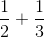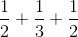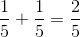SSAT Middle Level Math : How to add fractions

Example Questions

Example Question #1 : Build Fractions From Unit Fractions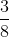is equal to which of the options below?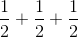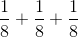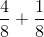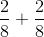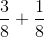Explanation:

When we add fractions, we have to have common denominators and we only add the numerators.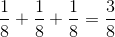Example Question #1 : Build Fractions From Unit Fractions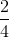is equal to which of the options below?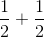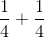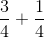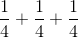Explanation:

When we add fractions, we have to have common denominators and we only add the numerators.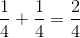Example Question #3 : Build Fractions From Unit Fractionsis equal to which of the options below?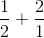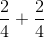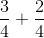Explanation:

When we add fractions, we have to have common denominators and we only add the numerators.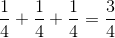Example Question #5 : Build Fractions From Unit Fractionsis equal to which of the options below?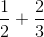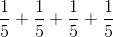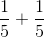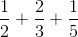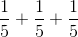Explanation:

When we add fractions, we have to have common denominators and we only add the numerators.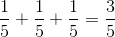Example Question #7 : Build Fractions From Unit Fractionsis equal to which of the options below?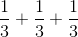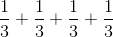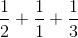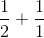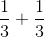Explanation:

When we add fractions, we have to have common denominators and we only add the numerators.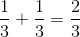Example Question #2 : Build Fractions From Unit Fractions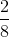is equal to which of the options below?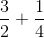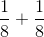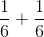Explanation:

When we add fractions, we have to have common denominators and we only add the numerators.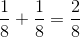Example Question #9 : Build Fractions From Unit Fractions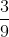is equal to which of the options below?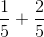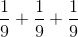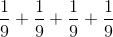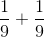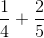Explanation:

When we add fractions, we have to have common denominators and we only add the numerators.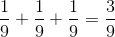Example Question #10 : Build Fractions From Unit Fractions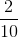is equal to which of the options below?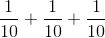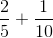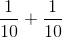Explanation:

When we add fractions, we have to have common denominators and we only add the numerators.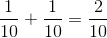Example Question #1421 : Ssat Middle Level Quantitative (Math)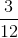is equal to which of the options below?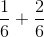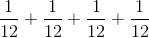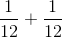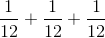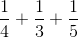Explanation:

When we add fractions, we have to have common denominators and we only add the numerators.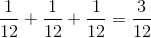Example Question #11 : Decompose A Fraction Into A Sum Of Fractions: Ccss.Math.Content.4.Nf.B.3bis equal to which of the options below?